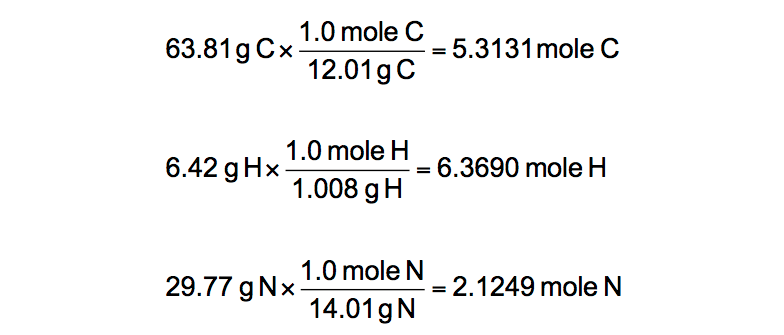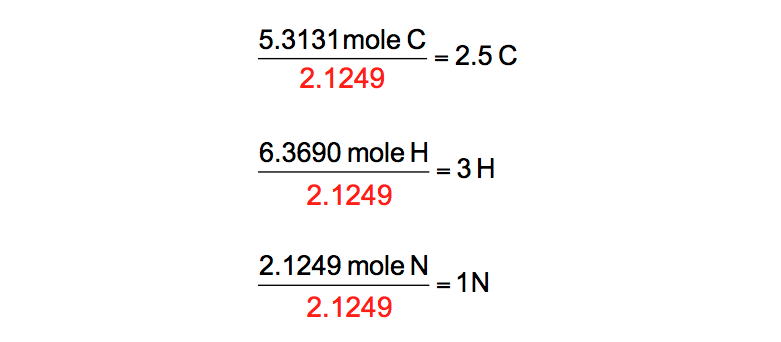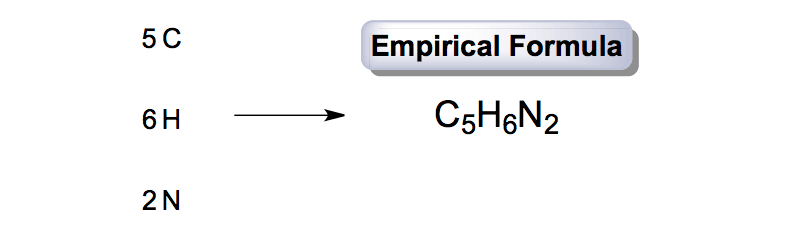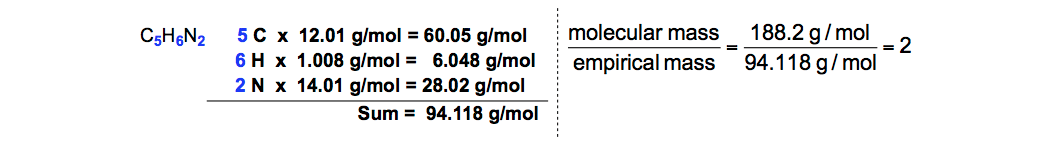Ch.3 - Chemical ReactionsWorksheetSee all chapters
 Ch.1 - Intro to General Chemistry 2hrs & 53mins 0% complete Worksheet Ch.2 - Atoms & Elements 2hrs & 40mins 0% complete Worksheet Ch.3 - Chemical Reactions 3hrs & 25mins 0% complete Worksheet BONUS: Lab Techniques and Procedures 1hr & 38mins 0% complete Worksheet BONUS: Mathematical Operations and Functions 47mins 0% complete Worksheet Ch.4 - Chemical Quantities & Aqueous Reactions 3hrs & 30mins 0% complete Worksheet Ch.5 - Gases 3hrs & 47mins 0% complete Worksheet Ch.6 - Thermochemistry 2hrs & 28mins 0% complete Worksheet Ch.7 - Quantum Mechanics 2hrs & 35mins 0% complete Worksheet Ch.8 - Periodic Properties of the Elements 1hr & 57mins 0% complete Worksheet Ch.9 - Bonding & Molecular Structure 2hrs & 5mins 0% complete Worksheet Ch.10 - Molecular Shapes & Valence Bond Theory 1hr & 31mins 0% complete Worksheet Ch.11 - Liquids, Solids & Intermolecular Forces 3hrs & 40mins 0% complete Worksheet Ch.12 - Solutions 2hrs & 17mins 0% complete Worksheet Ch.13 - Chemical Kinetics 2hrs & 22mins 0% complete Worksheet Ch.14 - Chemical Equilibrium 2hrs & 26mins 0% complete Worksheet Ch.15 - Acid and Base Equilibrium 4hrs & 42mins 0% complete Worksheet Ch.16 - Aqueous Equilibrium 3hrs & 48mins 0% complete Worksheet Ch. 17 - Chemical Thermodynamics 1hr & 44mins 0% complete Worksheet Ch.18 - Electrochemistry 2hrs & 58mins 0% complete Worksheet Ch.19 - Nuclear Chemistry 1hr & 33mins 0% complete Worksheet Ch.20 - Organic Chemistry 3hrs 0% complete Worksheet Ch.22 - Chemistry of the Nonmetals 2hrs & 1min 0% complete Worksheet Ch.23 - Transition Metals and Coordination Compounds 1hr & 54mins 0% complete Worksheet

# Empirical Formula

See all sectionsJules Bruno

The empirical formula represents the simplest whole numbered ratio of atoms in a compound, while the molecular formula or chemical formula represents the actual ratio of atoms in a compound.

How do you determine the empirical formula?

Questions usually begin with the percent compositions of elements to help find the empirical formula for ionic compounds or covalent compounds.

For example:

"A compound is composed of 63.81% carbon, 6.42% hydrogen, and 29.77% nitrogen by mass. Determine the empirical formula of this compound."

STEP 1: Whenever percentages are given we assume that they are out of 100 grams of compound. This allows us to automatically change percentages into grams.Percentages to grams conversion

STEP 2: Convert the grams of each element into moles.Grams to moles conversion

To avoid potential rounding errors, make sure you keep at least 3 decimal places for your answers in moles.

STEP 3: Divide all your mole answers by the smallest mole value calculated. Once you’ve divided all the moles by the smallest mole value you should obtain whole numbers.Ratio of Atomic Symbols

STEP 4: From division we have 2.5 carbons. This step only occurs when you don’t obtain whole numbers for each element.

We can only round if your value is X.1 or X.9. For example, if you had 2.1 carbons you could round down to 2 carbons. To obtain whole numbers we must multiply all the previous values by the same integer.Determining Whole Numbers

STEP 5: With whole numbers representing subscripts for each element we can now obtain the empirical formula.Empirical Formula

At this point we have our empirical formula. The additional step that follows is used when we are asked to determine the molecular formula.

STEP 6: For example:

"If the molar mass of the compound is 188.2 g/mol, what would be the molecular formula of the compound?"

To calculate the molecular formula we use the ratio below:Molecular Mass to Empirical Mass Ratio

The molecular mass or molar mass of the compound is traditionally given within the question, while the empirical mass is calculated from the empirical formula.Calculating Molecular Mass to Empirical Mass Ratio

Plugging in the molecular mass and empirical mass into the ratio will provide us with a whole number integer. To determine the molecular formula you will multiply the empirical formula by that integer.Molecular Formula

Beyond calculating the empirical and molecular formulas you can also deal with steps involving combustion analysisJules Bruno

Jules felt a void in his life after his English degree from Duke, so he started tutoring in 2007 and got a B.S. in Chemistry from FIU. He’s exceptionally skilled at making concepts dead simple and helping students in covalent bonds of knowledge.